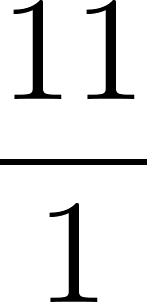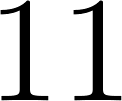×
Get Full Access to A Transition To Advanced Mathematics - 7 Edition - Chapter 1.1 - Problem 1
Get Full Access to A Transition To Advanced Mathematics - 7 Edition - Chapter 1.1 - Problem 1

×

# Use your knowledge of number systems to determine whether each is true orfalse.(a) 11 isISBN: 9780495562023 335

## Solution for problem 1 Chapter 1.1

A Transition to Advanced Mathematics | 7th Edition

• Textbook Solutions
• 2901 Step-by-step solutions solved by professors and subject experts
• Get 24/7 help from StudySoup virtual teaching assistantsA Transition to Advanced Mathematics | 7th Edition

4 5 1 251 Reviews
29
1
Problem 1

Use your knowledge of number systems to determine whether each is true orfalse.(a) 11 is a rational number. (b)5 is a rational number.(c) There are exactly 3 prime numbers between 40 and 50.(d) There are exactly 5 prime numbers less than 10.(e) 29 is a composite number.(f) 0 is a natural number. (g)(5 + 2i)(5 2i) is a real number.(h) 18 is a multiple of 12.

Step-by-Step Solution:

Problem 1

Use your knowledge of number systems to determine whether each is true or false. (a) 11 is a rational number. (b) 5 is a rational number. (c) There are exactly 3 prime numbers between 40 and 50. (d) There are exactly 5 prime numbers less than 10. (e) 29 is a composite number. (f) 0 is a natural number. (g) (5 + 2i)(5 2i) is a real number. (h) 18 is a multiple of 12.

Step-by-step solution

Step 1 of  8

a)A rational number that can be expressed as a simple fraction.

Write 11 in the fractional form asThus,is a rational number.

Hence,the given statement is true.

Step 2 of 8

Step 3 of 8

##### ISBN: 9780495562023

The answer to “Use your knowledge of number systems to determine whether each is true orfalse.(a) 11 is a rational number. (b)5 is a rational number.(c) There are exactly 3 prime numbers between 40 and 50.(d) There are exactly 5 prime numbers less than 10.(e) 29 is a composite number.(f) 0 is a natural number. (g)(5 + 2i)(5 2i) is a real number.(h) 18 is a multiple of 12.” is broken down into a number of easy to follow steps, and 66 words. This full solution covers the following key subjects: . This expansive textbook survival guide covers 39 chapters, and 619 solutions. The full step-by-step solution to problem: 1 from chapter: 1.1 was answered by , our top Math solution expert on 03/05/18, 08:54PM. Since the solution to 1 from 1.1 chapter was answered, more than 383 students have viewed the full step-by-step answer. This textbook survival guide was created for the textbook: A Transition to Advanced Mathematics, edition: 7. A Transition to Advanced Mathematics was written by and is associated to the ISBN: 9780495562023.

Unlock Textbook Solution